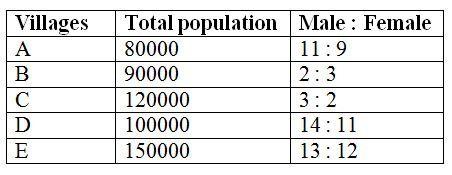# IBPS RRB PO Prelims Quantitative Aptitude Questions 2019 (Day-02)

Dear Aspirants, Our IBPS Guide team is providing new series of Quantitative Aptitude Questions for IBPS RRB PO Prelims 2019 so the aspirants can practice it on a daily basis. These questions are framed by our skilled experts after understanding your needs thoroughly. Aspirants can practice these new series questions daily to familiarize with the exact exam pattern and make your preparation effective.

Check here for IBPS RRB Prelims Mock Test 2019

[WpProQuiz 6515]

Directions (Q. 1 – 5): What value should come in place of question mark (?) in the following number series?

1) 1290, 645, 430, ? , 258, 215

a) 322.5

b) 356

c) 334

d) 342.5

e) 308

2) 218, 270, 333, 409, 500, ?

a) 712

b) 686

c) 730

d) 608

e) 654

3) 52, 274, 404, 472, 502, ?

a) 536

b) 512

c) 554

d) 580

e) 608

4) 11, 22, 66, ? , 2310, 25410

a) 360

b) 420

c) 330

d) 450

e) 480

5) 126, 64, 72, 171, ? , 6109

a) 652

b) 576

c) 924

d) 1032

e)748

Directions (Q. 6 – 10): Study the following information carefully and answer the given questions:

The following table shows the total population of 5 different villages and the ratio of males to females among them.6) Find the ratio between the total female population in village A to that of village C?

a) 5 : 6

b) 7 : 8

c) 1 : 2

d) 3 : 4

e) None of these

7)Total population in village B and C together is what percentage of total population in village D and E together?

a) 72 %

b) 76 %

c) 84 %

d) 88 %

e) None of these

8) Find the difference between the total population in village A to that of total male population in village B and E together?

a) 34000

b) 38000

c) 42000

d) 46000

e) None of these

9) Find the average male population in village B, C and E together?

a) 58000

b) 60000

c) 64000

d) 56000

e) None of these

10) Find the difference between the average male population of village B, C and E together to that of the average female population of village A, C and E together?

a)14000

b) 10000

c) 16000

d) 12000

e) None of these

Directions (1-5):

1290*(1/2) = 645

645*(2/3) = 430

430*(3/4) = 322.5

322.5*(4/5) = 258

258*(5/6) = 215

The difference of difference is, 11, 13, 15, 17,…

52 + 63 + 6 = 274

274 + 53 + 5 = 404

404 + 43 + 4 = 472

472 + 33 + 3 = 502

502 + 23 + 2 = 512

1*2 = 22

22*3 = 66

66*5 = 330

330*7 = 2310

2310*11 = 25410

126*0.5 + 13 = 64

64*1 + 23 = 72

72*2 + 33 = 171

171*4 +43 = 748

748*8 + 53 = 6109

Directions (6-10):

The total female population in village A

= >80000*(9/20) = 36000

The total female population in village C

= >120000*(2/5) = 48000

Required ratio = 36000 : 48000 = 3 : 4

Total population in village B and C together

= >90000 + 120000 = 210000

Total population in village D and E together

= >100000 + 150000 = 250000

Required % = (210000/250000)*100 = 84 %

The total population in village A = 80000

The total male population in village B and E together

= >[90000*(2/5)] + [150000*(13/25)]

= >36000 + 78000 = 114000

Required difference = 114000 – 80000 = 34000

The average male population in village B, C and E together

= >[90000*(2/5) + 120000*(3/5) + 150000*(13/25)]/3

= > [36000 + 72000 + 78000]/3

= > 62000

The average male population of village B, C and E together

= >[90000*(2/5) + 120000*(3/5) + 150000*(13/25)]/3

= > [36000 + 72000 + 78000]/3

= > 62000

The average female population of village A, C and E together

= >[80000*(9/20) + 120000*(2/5) + 150000*(12/25)]/3

= > [36000 + 48000 + 72000]/3

= > 52000

Required difference = 62000 – 52000 = 10000